## 4. Using the Solow Growth Model ¶

### 4.1. Convergence to the Balanced-Growth Path ¶

#### 4.1.1. The Example of Post-WWII West Germany ¶

Economies do converge to and then remain on their balanced-growth paths. The West German economy after World War II is a case in point.

We can see such convergence in action in many places and times. For example, consider the post-World War II history of West Germany. The defeat of the Nazis left the German economy at the end of World War II in ruins. Output per worker was less than one-third of its prewar level. The economy’s capital stock had been wrecked and devastated by three years of American and British bombing and then by the ground campaigns of the last six months of the war. But in the years immediately after the war, the West German economy’s capital-output ratio rapidly grew and converged back to its prewar value. Within 12 years the West German economy had closed half the gap back to its pre-World War II growth path. And within 30 years the West German economy had effectively closed the entire gap between where it had started at the end of World War II and its balanced-growth path.

In :
# bring in libraries needed for python

import pandas as pd
import numpy as np
import matplotlib.pyplot as plt

# load data from the penn world table's estimates
# of growth among the g-7 economies
#
# (these estimates have problems; but all estimates
# have problems, and a lot of work has gone into
# making these)


In :
# pull america out of the loaded data table

is_America = pwt91_df['countrycode'] == 'USA'
America_df = pwt91_df[is_America]
America_gdp_df = America_df[['year', 'rgdpna', 'emp']]
America_gdp_df['rgdpw'] = America_gdp_df.rgdpna/America_gdp_df.emp
America_pwg_ser = America_gdp_df[['year', 'rgdpw']]
America_pwg_ser.set_index('year',  inplace=True)

/Users/delong1/opt/anaconda3/lib/python3.7/site-packages/ipykernel_launcher.py:6: SettingWithCopyWarning:
A value is trying to be set on a copy of a slice from a DataFrame.
Try using .loc[row_indexer,col_indexer] = value instead

See the caveats in the documentation: http://pandas.pydata.org/pandas-docs/stable/user_guide/indexing.html#returning-a-view-versus-a-copy


In :
# pull germany out of the loaded data table, and
# plot growth

is_Germany = pwt91_df['countrycode'] == 'DEU'
Germany_df = pwt91_df[is_Germany]
Germany_gdp_df = Germany_df[['year', 'rgdpna', 'emp']]
Germany_gdp_df['rgdpw'] = Germany_gdp_df.rgdpna/Germany_gdp_df.emp
Germany_pwg_ser = Germany_gdp_df[['year', 'rgdpw']]
Germany_pwg_ser.set_index('year',  inplace=True)
np.log(Germany_pwg_ser).plot()
plt.show()

/Users/delong1/opt/anaconda3/lib/python3.7/site-packages/ipykernel_launcher.py:7: SettingWithCopyWarning:
A value is trying to be set on a copy of a slice from a DataFrame.
Try using .loc[row_indexer,col_indexer] = value instead

See the caveats in the documentation: http://pandas.pydata.org/pandas-docs/stable/user_guide/indexing.html#returning-a-view-versus-a-copy
import sys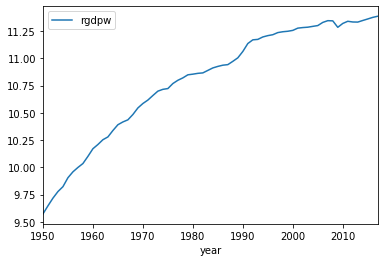In :
# compare german real national income per worker
# with american

Germany_ratio_ser = Germany_pwg_ser/America_pwg_ser
Germany_ratio_ser.plot()
plt.show()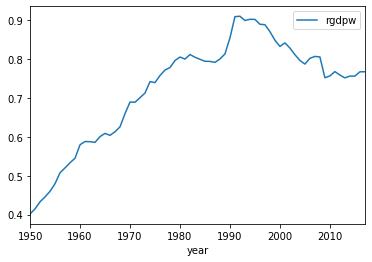The two figures above show, respectively, the natural logarithm of absolute real national income per worker for the German economy and real national income per worker relative to the U.S. value, both since 1950. By 1980 the German economy had converged: its period of rapid recovery growth was over, and national income per capita then grew at the same rate as that in the U.S., which had not suffered wartime destruction pushing it off and below its steady-state balanced-growth path. Then in 1990, at least according to this set of estimates, the absorption of the formerly communist East German state into the Bundesrepublik was an enormous benefit: the expanded division of labor and return of the market economy allowed productivity in the German east to more than double almost overnight. Thereafter the German economy has lost some ground relative to the U.S. as the U.S.'s leading information technology hardware and software sectors have been much stronger leading sectors than Germany's precision machinery and manufacturing sectors.

By comparison, the United States shows no analogous period of rapid growth catching up to a steady-state balanced-growth path. (There is, however, a marked boom in the 1960s, and then a return to early trends in the late 1970s and 1980s, followed by a return to previous normal growth in the 1990s and then a fall-off in growth after 2007.)

In :
# plot american growth since 1950

np.log(America_pwg_ser).plot()
plt.show()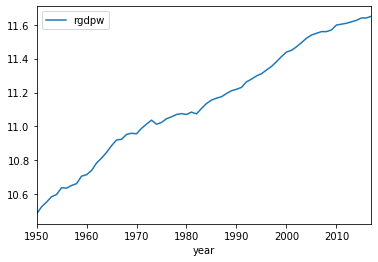#### 4.1.2. The Example of Post-WWII Japan ¶

The same story holds in an even stronger form for the other defeated fascist power that surrendered unconditionally to the U.S. at the end of World War II.

In 1950, largely as a result of Curtis LeMay's B-29s, Japan is only half as productive as Germany, and only one-fifth as productive as the United States. Once again, it converges rapidly. After 1990 Japan no longer grows faster than and catches up to the United States. Indeed, like Germany it thereafter loses ground as its world class manufacturing sectors are also less powerful leading sectors than the United States's information technology hardware and software complexes.

In :
# pull japan out of the loaded data table, and
# plot growth

is_Japan = pwt91_df['countrycode'] == 'JPN'
Japan_df = pwt91_df[is_Japan]
Japan_gdp_df = Japan_df[['year', 'rgdpna', 'emp']]
Japan_gdp_df['rgdpw'] = Japan_gdp_df.rgdpna/Japan_gdp_df.emp
Japan_pwg_ser = Japan_gdp_df[['year', 'rgdpw']]
Japan_pwg_ser.set_index('year',  inplace=True)
np.log(Japan_pwg_ser).plot()
plt.show()

/Users/delong1/opt/anaconda3/lib/python3.7/site-packages/ipykernel_launcher.py:7: SettingWithCopyWarning:
A value is trying to be set on a copy of a slice from a DataFrame.
Try using .loc[row_indexer,col_indexer] = value instead

See the caveats in the documentation: http://pandas.pydata.org/pandas-docs/stable/user_guide/indexing.html#returning-a-view-versus-a-copy
import sys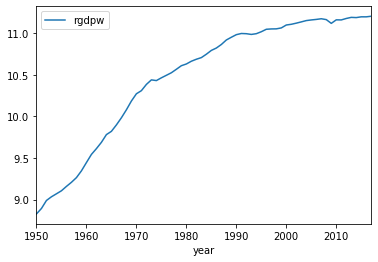In :
# compare japan to america

Japan_ratio_ser = Japan_pwg_ser/America_pwg_ser
Japan_ratio_ser.plot()
plt.show()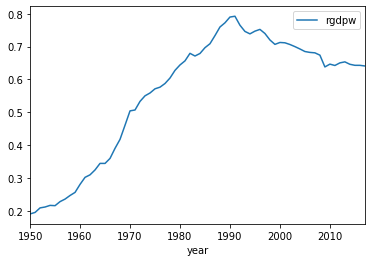#### 4.1.3. The Post-WWII G-7 ¶

The same story holds for the other members of the G-7 group of large advanced industrial economies as well.

In :
# pull britain out of the loaded data table

is_Britain = pwt91_df['countrycode'] == 'GBR'
Britain_df = pwt91_df[is_Britain]
Britain_gdp_df = Britain_df[['year', 'rgdpna', 'emp']]
Britain_gdp_df['rgdpw'] = Britain_gdp_df.rgdpna/Britain_gdp_df.emp
Britain_pwg_ser = Britain_gdp_df[['year', 'rgdpw']]
Britain_ratio_ser = Britain_pwg_ser/America_pwg_ser
Britain_pwg_ser.set_index('year',  inplace=True)

/Users/delong1/opt/anaconda3/lib/python3.7/site-packages/ipykernel_launcher.py:6: SettingWithCopyWarning:
A value is trying to be set on a copy of a slice from a DataFrame.
Try using .loc[row_indexer,col_indexer] = value instead

See the caveats in the documentation: http://pandas.pydata.org/pandas-docs/stable/user_guide/indexing.html#returning-a-view-versus-a-copy


In :
# pull italy out of the loaded data table

is_Italy = pwt91_df['countrycode'] == 'ITA'
Italy_df = pwt91_df[is_Italy]
Italy_gdp_df = Italy_df[['year', 'rgdpna', 'emp']]
Italy_gdp_df['rgdpw'] = Italy_gdp_df.rgdpna/Italy_gdp_df.emp
Italy_pwg_ser = Italy_gdp_df[['year', 'rgdpw']]
Italy_ratio_ser = Italy_pwg_ser/America_pwg_ser
Italy_pwg_ser.set_index('year',  inplace=True)

/Users/delong1/opt/anaconda3/lib/python3.7/site-packages/ipykernel_launcher.py:6: SettingWithCopyWarning:
A value is trying to be set on a copy of a slice from a DataFrame.
Try using .loc[row_indexer,col_indexer] = value instead

See the caveats in the documentation: http://pandas.pydata.org/pandas-docs/stable/user_guide/indexing.html#returning-a-view-versus-a-copy


In :
# pull canada out of the loaded data table


/Users/delong1/opt/anaconda3/lib/python3.7/site-packages/ipykernel_launcher.py:6: SettingWithCopyWarning:
A value is trying to be set on a copy of a slice from a DataFrame.
Try using .loc[row_indexer,col_indexer] = value instead

See the caveats in the documentation: http://pandas.pydata.org/pandas-docs/stable/user_guide/indexing.html#returning-a-view-versus-a-copy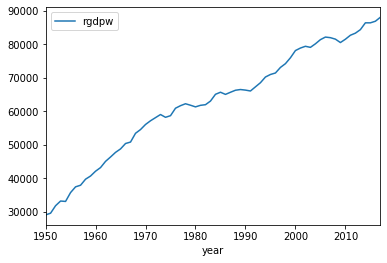In :
# pull italy out of the loaded data table

is_France = pwt91_df['countrycode'] == 'FRA'
France_df = pwt91_df[is_France]
France_gdp_df = France_df[['year', 'rgdpna', 'emp']]
France_gdp_df['rgdpw'] = France_gdp_df.rgdpna/France_gdp_df.emp
France_pwg_ser = France_gdp_df[['year', 'rgdpw']]
France_ratio_ser = France_pwg_ser/America_pwg_ser
France_pwg_ser.set_index('year',  inplace=True)

/Users/delong1/opt/anaconda3/lib/python3.7/site-packages/ipykernel_launcher.py:6: SettingWithCopyWarning:
A value is trying to be set on a copy of a slice from a DataFrame.
Try using .loc[row_indexer,col_indexer] = value instead

See the caveats in the documentation: http://pandas.pydata.org/pandas-docs/stable/user_guide/indexing.html#returning-a-view-versus-a-copy


In :
# plot g-7 natural logarithm levels of national
# income per worker

g7_df = pd.DataFrame()
g7_df['Japan'] = np.log(Japan_pwg_ser['rgdpw'])
g7_df['Germany'] = np.log(Germany_pwg_ser['rgdpw'])
g7_df['America'] = np.log(America_pwg_ser['rgdpw'])
g7_df['Italy'] = np.log(Italy_pwg_ser['rgdpw'])
g7_df['France'] = np.log(France_pwg_ser['rgdpw'])
g7_df['Britain'] = np.log(Britain_pwg_ser['rgdpw'])
g7_df.plot()
plt.show()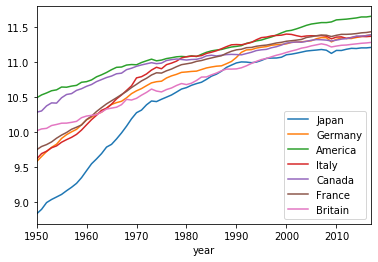In :
# calculate and plot g-7 levels of national income
# per worker as a proportion of american

g7_ratio_df = pd.DataFrame()
g7_ratio_df['Japan'] = Japan_pwg_ser['rgdpw']/America_pwg_ser['rgdpw']
g7_ratio_df['Germany'] = Germany_pwg_ser['rgdpw']/America_pwg_ser['rgdpw']
g7_ratio_df['America'] = America_pwg_ser['rgdpw']/America_pwg_ser['rgdpw']
g7_ratio_df['Italy'] = Italy_pwg_ser['rgdpw']/America_pwg_ser['rgdpw']
g7_ratio_df['France'] = France_pwg_ser['rgdpw']/America_pwg_ser['rgdpw']
g7_ratio_df['Britain'] = Britain_pwg_ser['rgdpw']/America_pwg_ser['rgdpw']
g7_ratio_df.plot()
plt.show()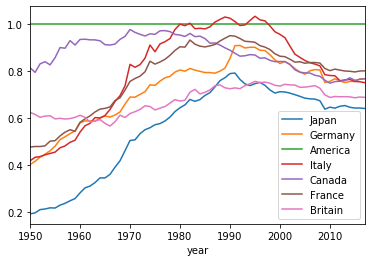The idea—derived from the Solow model—that economies pushed off and below their steady-state balanced-growth paths by the destruction and chaos of war thereafter experience a period of supergrowth that ebbs as they approach their steady-state balanced-growth paths from below story holds for the other members of the G-7 group of large advanced industrial economies as well. In increasing order of the magnitude of their shortfall vis-a-vis the U.S. and the speed of recovery supergrowth, we have: France, Italy, Germany, and Japan. The three economies that escaped wartime chaos and destruction—the U.S., Britain, and Canada—do not exhibit supergrowth until catchup to their steady-state balanced-growth paths.

There is a lot more going on in the post-WWII history of the G-7 economies than just catchup to their steady-state balanced-growth paths after the destruction of World War II: Why do the other economies lose ground vis-a-vis the U.S. after 1990? Why does the U.S. exhibit a small speedup, slowdown, speedup, and then renewed slowdown again? What is it with Britain's steady-state balanced-growth path having so much lower productivity than the other Europeans? Why is Japan the most different from its G-7 partners? And what is it with Italy's attaining U.S. worker productivity levels in 1980, and then its post-2000 relative collapse? (The post-2000 collapse in Italian growth is real; the estimate that it was as productive as the U.S. from 1980-2000 is a data construction error.)

### 4.2. Analyzing Jumps in Parameter Values ¶

What if one or more of the parameters in the Solow growth model were to suddenly and substantially shift? What if the labor-force growth rate were to rise, or the rate of technological progress to fall?

One principal use of the Solow growth model is to analyze questions like these: how changes in the economic environment and in economic policy will affect an economy’s long-run levels and growth path of output per worker Y/L.

Let’s consider, as examples, several such shifts: an increase in the growth rate of the labor force n, a change in the economy’s saving-investment rate s, and a change in the growth rate of labor efficiency g. All of these will have effects on the balanced- growth path level of output per worker. But only one—the change in the growth rate of labor efficiency—will permanently affect the growth rate of the economy.

We will assume that the economy starts on its balanced growth path—the old balanced growth path, the pre-shift balanced growth path. Then we will have one (or more) of the parameters—the savings-investment rate s, the labor force growth rate n, the labor efficiency growth rate g—jump discontinuously, and then remain at its new level indefinitely. The jump will shift the balanced growth path. But the level of output per worker will not immediately jump. Instead, the economy's variables will then, starting from their old balanced growth path values, begin to converge to the new balanced growth path—and converge in the standard way.

Remind yourselves of the key equations for understanding the model:

The level of output per worker is:

(4.1) $\frac{Y}{L} = \left( \frac{K}{Y} \right)^{\theta}E$

The balanced-growth path level of output per worker is:

(4.2) $\left( \frac{Y}{L} \right)^* = \left( \frac{s}{n+g+δ} \right)^{\theta}E$

The speed of convergence of the capital-output ratio to its balanced-growth path value is:

(4.3) $\frac{d(K/Y)}{dt} = −(1−α)(n+g+δ) \left[ \frac{K}{Y} − \frac{s}{(n+g+δ)} \right]$

where, you recall $\theta = \alpha/(1-\alpha)$ and $\alpha = \theta/(1+\theta)$

#### 4.2.1. A Shift in the Labor-Force Growth Rate ¶

Real-world economies exhibit profound shifts in labor-force growth. The average woman in India today has only half the number of children that the average woman in India had only half a century ago. The U.S. labor force in the early eighteenth century grew at nearly 3 percent per year, doubling every 24 years. Today the U.S. labor force grows at 1 percent per year. Changes in the level of prosperity, changes in the freedom of migration, changes in the status of women that open up new categories of jobs to them (Supreme Court Justice Sandra Day O’Connor could not get a private-sector legal job in San Francisco when she graduated from Stanford Law School even with her amazingly high class rank), changes in the average age of marriage or the availability of birth control that change fertility—all of these have powerful effects on economies’ rates of labor-force growth.

What effects do such changes have on output per worker Y/L—on our mea sure of material prosperity? The faster the growth rate of the labor force n, the lower will be the economy’s balanced-growth capital-output ratio s/(n + g - δ). Why? Because each new worker who joins the labor force must be equipped with enough capital to be productive and to, on average, match the productivity of his or her peers. The faster the rate of growth of the labor force, the larger the share of current investment that must go to equip new members of the labor force with the capital they need to be productive. Thus the lower will be the amount of invest ment that can be devoted to building up the average ratio of capital to output.

A sudden and permanent increase in the rate of growth of the labor force will lower the level of output per worker on the balanced-growth path. How large will the long-run change in the level of output be, relative to what would have happened had labor-force growth not increased? It is straightforward to calculate if we know the other parameter values, as is shown in the example below.

4.2.1.1. An Example: An Increase in the Labor Force Growth Rate: Consider an economy in which the parameter α is 1/2, the efficiency of labor growth rate g is 1.5 percent per year, the depreciation rate δ is 3.5 percent per year, and the saving rate s is 21 percent. Suppose that the labor-force growth rate suddenly and permanently increases from 1 to 2 percent per year.

Before the increase in the labor-force growth rate, in the initial steady-state, the balanced-growth equilibrium capital-output ratio was:

(4.4) $\left( \frac{K_{in}}{Y_{in}} \right)^* = \frac{s_{in}}{(n_{in}+g_{in}+δ_{in})} = \frac{0.21}{(0.01 + 0.015 + 0.035)} = \frac{0.21}{0.06} = 3.5$

(with subscripts "in" for "initial).

After the increase in the labor-force growth rate, in the alternative steady state, the new balanced-growth equilibrium capital-output ratio will be:

(4.5) $\left( \frac{K_{alt}}{Y_{alt}} \right)^* = \frac{s_{alt}}{(n_{alt}+g_{alt}+δ_{alt})} = \frac{0.21}{(0.02 + 0.015 + 0.035)} = \frac{0.21}{0.07} = 3$

(with subscripts "alt" for "alternative").

Before the increase in labor-force growth, the level of output per worker along the balanced-growth path was equal to:

(4.6) $\left( \frac{Y_{t, in}}{L_{t, in}} \right)^* = \left( \frac{s_{in}}{(n_{in}+g_{in}+δ_{in})} \right)^{α/(1−α)} E_{t, in} = 3.5 E_{t, in}$

After the increase in labor-force growth, the level of output per worker along the balanced-growth path will be equal to:

(4.7) $\left( \frac{Y_{t, alt}}{L_{t, alt}} \right)^* = \left( \frac{s_{alt}}{(n_{alt}+g_{alt}+δ_{alt})} \right)^{α/(1−α)} E_{t, alt} = 3 E_{t, alt}$

This fall in the balanced-growth path level of output per worker means that in the long run—after the economy has converged to its new balanced-growth path—one-seventh of its per worker economic prosperity has been lost because of the increase in the rate of labor-force growth.

In the short run of a year or two, however, such an increase in the labor-force growth rate has little effect on output per worker. In the months and years after labor-force growth increases, the increased rate of labor-force growth has had no time to affect the economy’s capital-output ratio. But over decades and generations, the capital-output ratio will fall as it converges to its new balanced-growth equilibrium level.

A sudden and permanent change in the rate of growth of the labor force will immediately and substantially change the level of output per worker along the economy’s balanced-growth path: It will shift the balanced-growth path for output per worker up (if labor-force growth falls) or down (if labor-force growth rises). But there is no corresponding immediate jump in the actual level of output per worker in the economy. Output per worker doesn’t immediately jump—it is just that the shift in the balanced-growth path means that the economy is no longer in its Solow growth model long-run equilibrium.

4.2.1.2. Empirics: The Labor-Force Growth Rate Matters: The average country with a labor-force growth rate of less than 1 percent per year has an output-per-worker level that is nearly 60 percent of the U.S. level. The average country with a labor-force growth rate of more than 3 percent per year has an output-per-worker level that is only 20 percent of the U.S. level.

To some degree poor countries have fast labor-force growth rates because they are poor: Causation runs both ways. Nevertheless, high labor-force growth rates are a powerful cause of low capital intensity and relative poverty in the world today.

Figure 4.2.1: The Labor Force Growth Rate Matters: Output per Worker and Labor Force GrowthHow important is all this in the real world? Does a high rate of labor-force growth play a role in making countries relatively poor not just in economists’ models but in reality? It turns out that it is important. Of the 22 countries in the world in 2000 with output-per-worker levels at least half of the U.S. level, 18 had labor-force growth rates of less than 2 percent per year, and 12 had labor-force growth rates of less than 1 percent per year. The additional investment requirements imposed by rapid labor-force growth are a powerful reducer of capital intensity and a powerful obstacle to rapid economic growth.

It takes time, decades and generations, for the economy to converge to its new balanced-growth path equilibrium, and thus for the shift in labor-force growth to affect average prosperity and living standards. But the time needed is reason for governments that value their countries’ long-run prosperity to take steps now (or even sooner) to start assisting the demographic transition to low levels of population growth. Female education, social changes that provide women with more opportunities than being a housewife, inexpensive birth control—all these pay large long-run dividends as far as national prosperity levels are concerned.

U.S. President John F Kennedy used to tell a story of a retired French general, Marshal Lyautey, “who once asked his gardener to plant a tree. The gardener objected that the tree was slow-growing and would not reach maturity for a hundred years. The Marshal replied, ‘In that case, there is no time to lose, plant it this afternoon.’”

#### 4.2.2. The Algebra of a Higher Labor Force Growth Rate ¶

But rather than calculating example by example, set of parameter values by set of parameter values, we can gain some insight by resorting to algebra, and consider in generality the effect on capital-output ratios and output per worker levels of an increase Δn in the labor force growth rate, following an old math convention of using "Δ" to stand for a sudden and discrete change.

Assume the economy has its Solow growth parameters, and its initial balanced-growth path capital-output ratio

(4.8) $\left( \frac{K_{in}}{Y_{in}} \right)^* = \frac{s_{in}}{(n_{in}+g_{in}+δ_{in})}$

with "in" standing for "initial".

And now let us consider an alternative scenario, with "alt" standing for "alternative", in which things had been different for a long time:

(4.9) $\left( \frac{K_{alt}}{Y_{alt}} \right)^* = \frac{s_{alt}}{(n_{alt}+g_{alt}+δ_{alt})}$

For the g and δ parameters, their initial values are their alternative values. And for the labor force growth rate:

(4.10) $n_{alt} = n_{in} + Δn$

So we can then rewrite:

(4.11) $\left( \frac{K_{alt}}{Y_{alt}} \right)^* = \frac{s_{in}}{(n_{in}+g_{in}+δ_{in})} \frac{(n_{in}+g_{in}+δ_{in})}{(n_{in} + Δn +g_{in}+δ_{in})} = \frac{s_{in}}{(n_{in}+g_{in}+δ_{in})} \left[\frac{1}{1+\frac{Δn}{(n_{in}+g_{in}+δ_{in})}} \right]$

The first term on the right hand side is just the initial capital-output ratio, and we know that 1/(1+x) is approximately 1−x for small values of x, so we can make an approximation:

(4.12) $\left( \frac{K_{alt}}{Y_{alt}} \right)^* = \left( \frac{K_{in}}{Y_{in}} \right)^* \left[ 1 - \frac{Δn}{(n_{in}+g_{in}+δ_{in})} \right]$

Take the proportional change in the denominator (n+g+δ) of the expression for the balanced-growth capital-output ratio. Multiply that proportional change by the initial balanced-growth capital-output ratio. That is the differential we are looking for.

And by amplifying or damping that change by raising to the α/(1−α) power, we get the differential for output per worker.

#### 4.2.3. A Shift in the Growth Rate of the Efficiency of Labor ¶

4.2.3.1. Efficiency of Labor the Master Key to Long Run Growth: By far the most important impact on an economy’s balanced-growth path values of output per worker, however, is from shifts in the growth rate of the efhciency of labor g. We already know that growth in the efhciency of labor is absolutely essential for sustained growth in output per worker and that changes in g are the only things that cause permanent changes in growth rates that cumulate indehnitely.

Recall yet one more time the capital-output ratio form of the production function:

(4.13) $\frac{Y}{L} = \left( \frac{K}{Y} \right)^{\theta} E$

Consider what this tells us. We know that a Solow growth model economy converges to a balanced-growth path. We know that the capital-output ratio K/Y is constant along the balanced-growth path. We know that the returns-to-investment parameter α is constant. And so the balanced-growth path level of output per worker Y/L grows only if, and grows only as fast as, the efficiency of labor E grows.

4.2.3.2. Efficiency of Labor Growth and the Capital-Output Ratio: Yet when we took a look at the math of an economy on its balanced growth path:

(4.14) $\left( \frac{Y}{L} \right)^* = \left( \frac{s}{n+g+δ} \right)^{\theta} E$

we also see that an increase in g raises the denominator of the first term on the right hand side—and so pushes the balanced-growth capital output ratio down. That implies that the balanced-growth path level of output per worker associated with any level of the efficiency of labor down as well.

It is indeed the case that—just as in the case of an increased labor force growth rate n—an increased efficiency-of-labor growth rate g reduces the economy’s balanced-growth capital-output ratio s/(n + g - δ). Why? Because, analogously with an increase in the labor force, increases in the efficiency of labor allow each worker to do the work of more, but they need the machines and buildings to do them. The faster the rate of growth of the efficiency of la or, the larger the share of current investment that must go to keep up with the rising efficiency of old members of the labor force and supply them with the capital they need to be productive. Thus the lower will be the amount of investment that can be devoted to building up or maintaining the average ratio of capital to output.

#### 4.2.4. The Algebra of Shifting the Efficiency-of-Labor Growth Rate ¶

The arithmetic and algebra are, for the beginning and the middle, the same as they were for an increase in the rate of labor force growth:

Assume the economy has its Solow growth parameters, and its initial balanced-growth path capital-output ratio:

(4.15) $\left( \frac{K_{in}}{Y_{in}} \right)^* = \frac{s}{(n_+g_{in}+δ)}$

(with "in" standing for "initial"). Also consider an alternative scenario, with "alt" standing for "alternative", in which things had been different for a long time, with a higher efficiency-of-labor growth rate g+Δg since some time t=0 now far in the past:

(4.16) $\left( \frac{K_{alt}}{Y_{alt}} \right)^* = \frac{s}{(n+g+Δg+δ)}$

We can rewrite this as:

(4.17) $\left( \frac{K_{alt}}{Y_{alt}} \right)^* =$ $\frac{s}{(n+g_{in}+δ)} \frac{(n+g_{in}+δ)}{(n +g_{in}+Δg+δ)} =$ $\frac{s}{(n+g_{in}+δ)} \left[\frac{1}{1+\frac{Δg}{(n+g_{in}+δ)}} \right]$

Once again, the first term on the right hand side is just the initial capital-output ratio, and we know that 1/1+x is approximately 1−x for small values of x, so we can make an approximation:

(4.18) $\left( \frac{K_{alt}}{Y_{alt}} \right)^* = \left( \frac{K_{in}}{Y_{in}} \right)^* \left[ 1 - \frac{Δg}{(n+g_{in}+δ)} \right]$

Take the proportional change in the denominator of the expression for the balanced-growth capital output ratio. Multiply that proportional change by the initial balanced-growth capital-output ratio. That is the differential in the balanced-growth capital-output ratio that we are looking for.

But how do we translate that into a differential for output per worker? In the case of an increase in the labor force growth rate, it was simply by amplifying or damping the change in the balanced-growth capital-output ratio by raising it to the power $\theta = (α/(1−α))$ in order to get the differential for output per worker. We could do that because the efficiency-of-labor at every time t $E_t$ was the same in both the initial and the alternative scenarios.

That is not the case here.

Here, the efficiency of labor was the same in the initial and alternative scenarios back at time 0, now long ago. Since then E has been growing at its rate g in the initial scenario, and at its rate g+Δg in the alternative scenario, and so the time subscripts will be important. Thus for the alternative scenario:

(4.19) $\left( \frac{Y_{t, alt}}{L_{t, alt}} \right)^*$ $\left( \frac{s}{(n+g_{in} + \Delta g + \delta)} \right) ^{\theta)}(1+(g_{in}+ \Delta g))^t E_0$

while for the initial scenario:

(4.20) $\left( \frac{Y_{t, ini}}{L_{t, ini}} \right)^*$ $\left( \frac{s}{(n+g_{in} + \delta)} \right) ^{\theta}$ $(1+g_{in})^t E_0$

Now divide to get the ratio of output per worker under the alternative and initial scenarios:

(4.21) $\left( \frac{Y_{t, alt}/L_{t, alt}}{Y_{t, ini}/L_{t, ini}} \right)^* = \left( \frac{n+g_{in}+\delta}{(n+g_{in}+\Delta g + \delta)} \right)^{\theta} (1+ \Delta g)^t$

Thus we see that in the long run, as the second term on the right hand side compounds as t grows, balanced-growth path output per worker under the alternative becomes first larger and then immensely larger than output per worker under the initial scenario. Yes, the balanced-growth path capital-output ratio is lower. But the efficiency of labor at any time t is higher, and then vastly higher if Δgt has had a chance to mount up and thus (1+Δg)t has had a chance to compound.

Yes, a positive in the efficiency of labor growth g does reduce the economy’s balanced-growth path capital-output ratio. But these effects are overwhelmed by the more direct effect ofa larger g on output per worker. It is the economy with a high rate of efficiency of labor force growth g that becomes by far the richest over time. This is our most important conclusion. In the very longest run, the growth rate of the standard of living—of output per worker—can change if and only if the growth rate of labor efficiency changes. Other factors—a higher saving-investment rate, lower labor-force growth rate, or lower depreciation rate—can and down. But their effects are short and medium effects: They do not permanently change the growth rate of output per worker, because after the economy has converged to its balanced growth path the only determinant of the growth rate of output per worker is the growth rate of labor efficiency: both are equal to g.

Thus, if we are to increase the rate of growth of the standard of living permanently, we must pursue policies that increase the rate at which labor efficiency grows—policies that enhance technological and organizational progress, improve worker skills, and add to worker education.

4.2.4.1. An Example: Shifting the Growth Rate of the Efficiency of Labor: What are the effects of an increase in the rate of growth of the efficiency of labor? Let's work through an example:

Suppose we have, at some moment we will label time 0, t=0, an economy on its balanced growth path with a savings rate s of 20% per year, a labor force growth rate n or 1% per year, a depreciation rate δ of 3% per year, an efficiency-of-labor growth rate g of 1% per year, and a production function curvature parameter α of 1/2 and thus a $\theta = 1$. Suppose that at that moment t=0 the labor force $L_0$ is 150 million, and the efficiency of labor $E_0$ is 35000.

It is straightforward to calculate the economy at that time 0. Because the economy is on its balanced growth path, its capital-output ratio K/Y is equal to the balanced-growth path capital-output ratio (K/Y)*:

(4.22) $\frac{K_0}{Y_0} = \left( \frac{K}{Y} \right)^* = \frac{s}{n+g+\delta} = \frac{0.2}{0.01 + 0.01 + 0.03} = 4$

And with an efficiency of labor value $E_0=70000$, output per worker at time zero is:

(4.23) $\frac{Y_0}{L_0} = \left( \frac{K_0}{Y_0} \right)^{\theta} = 4^1 (35000) = 140000$

Since the economy is on its balanced growth path, the rate of growth of output per worker is equal to the rate of growth of efficiency per worker. Since the efficiency of labor is growing at 1% per year, we can calculate what output per worker would be at any future time t should the parameters describing the economy remain the same:

(4.24) $\left(\frac{Y_t}{L_t}\right)_ini = (140000)e^{0.01t}$

where the subscript "ini" tells us that this value belongs to an economy that retains its initial parameter values into the future. Thus 69 years into the future, at t=69:

(4.25) $\left(\frac{Y_69}{L_69}\right)_ini = (140000)e^{(0.01)(69)} = (140000)(1.9937) = 279120$

Now let us consider an alternative scenario in which output per worker is the same in year 0 but in which the efficiency of labor growth rate g is a higher rate. Suppose $g_{alt} = g_{ini} + Δg$, with the subscript "alt" reminding us that this parameter or variable belongs to the alternative scenario just as "ini" reminds us of the initial scenario or set of values. How do we forecast the growth of the economy in an alternative scenario—in this case, in an alternative scenario in which $Δg=0.02$

The first thing to do is to calculate the balanced growth path steady-state capital-output ratio in this alternative scenario. Thus we calculate:

(4.26) $\left( \frac{K}{Y} \right)_{alt}^* = \frac{s}{n + g_{ini} + Δg + δ} = \frac{0.20}{0.01 + 0.01 + 0.02 + 0. 03} = \frac{0.20}{0.07} = 2.857$

The steady-state balanced growth path capital-output ratio is much lower in the alternative scenario than it was in the initial scenario: 2.857 rather than 4. The capital-output ratio, of course, does not drop instantly to its new steady-state value. It takes time for the transition to occur.

While the transition is occurring, the efficiency of labor in the alternative scenario is growing at not 1% but 3% per year. We can thus calculate the alternative scenario balanced growth path value of output per worker as:

(4.27) $\left(\frac{Y_t}{L_t}\right)_{alt}^* = \left( \frac{K}{Y} \right)_{alt}^{*\theta} E_0 e^{(0.01+0.02)t}$

And in the 69th year this will be:

(4.28) $\left(\frac{Y_69}{L_69}\right)_{alt}^* = (2.857)(35000) e^{(0.03)(69)} = 792443$

How good would this balanced growth path value be as an estimate of the actual behavior of the economy? We know that a Solow growth model economy closes a fraction (1−α)(n+g+δ) of the gap between its current position and its steady-state balanced growth path capital-output ratio each period. For our parameter values (1−α)(n+g+δ)=0.035. That gives us about 20 years as the period needed to converge halfway to the balanced growth path. 69 years is thus about 3.5 such halvings of the gap—meaning that the economy will close 9/10 of the way. Thus assuming the economy is on its alternative scenario balanced growth path in year 69 is not a bad assumption.

But if we want to calculate the estimate exactly? 820752.

The takeaways are three:

For these parameter values, 69 years are definitely long enough for you to make the assumption that the economy has converged to its Solow model balanced growth path. One year no. Ten years no. Sixty-nine years, yes.

Shifts in the growth rate g of the efficiency of labor do, over time, deliver enormous differentials in output per worker across scenarios.

The higher efficiency of labor economy is, in a sense, a less capital intensive economy: only 2.959 years' worth of current production is committed to and tied up in the economy's capital stock in the alternative scenario, while 4 years' worth was tied up in the initial scenario. But the reduction in output per worker generated by a lower capital-output ratio is absolutely swamped by the faster growth of the efficiency of labor, and thus the much greater value of the efficiency of labor in the alternative scenario comes the 69th year.

#### 4.2.5. Shifts in the Saving Rate s ¶

4.2.5.1 The Most Common Policy and Environment Shock: Shifts in labor force growth rates do happen: changes in immigration policy, the coming of cheap and easy contraception (or, earlier, widespread female literacy), or increased prosperity and expected prosperity that trigger "baby booms" can all have powerful and persistent effects on labor force growth down the pike. Shifts in the growth of labor efficiency growth happen as well: economic policy disasters and triumphs, countless forecasted "new economies" and "secular stagnations", and the huge economic shocks that were the first and second Industrial Revolutions—the latter inaugurating that global era of previously unimagined increasing prosperity we call modern economic growth—push an economy's labor efficiency growth rate g up or down and keep it there.

Nevertheless, the most frequent sources of shifts in the parameters of the Solow growth model are shifts in the economy’s saving-investment rate. The rise of politicians eager to promise goodies—whether new spending programs or tax cuts — to voters induces large government budget deficits, which can be a persistent drag on an economy’s saving rate and its rate of capital accumulation. Foreigners become alternately overoptimistic and overpessimistic about the value of investing in our country, and so either foreign saving adds to or foreign capital flight reduces our own saving- investment rate. Changes in households’ fears of future economic disaster, in households’ access to credit, or in any of numerous other factors change the share of household income that is saved and invested. Changes in government tax policy may push after-tax returns up enough to call forth additional savings, or down enough to make savings seem next to pointless. Plus rational or irrational changes in optimism or pessimism—what John Maynard Keynes labelled the "animal spirits" of individual entrepreneurs, individual financiers, or bureaucratic committees in firms or banks or funds all can and do push an economy's savings-investment rate up and down.

4.2.5.2 Analyzing a Shift in the Saving Rate s: What effects do changes in saving rates have on the balanced-growth path levels of Y/L?

The higher the share of national product devoted to saving and gross investment—the higher is s—the higher will be the economy’s balanced-growth capital-output ratio s/(n + g + δ). Why? Because more investment increases the amount of new capital that can be devoted to building up the average ratio of cap ital to output. Double the share of national product spent on gross investment, and you will find that you have doubled the economy’s capital intensity, or its average ratio of capital to output.

As before, the equilibrium will be that point at which the economy’s savings effort and its investment requirements are in balance so that the capital stock and output grow at the same rate, and so the capital-output ratio is constant. The savings effort of society is simply sY, the amount of total output devoted to saving and investment. The investment requirements are the amount of new capital needed to replace depreciated and worn-out machines and buildings, plus the amount needed to equip new workers who increase the labor force, plus the amount needed to keep the stock of tools and machines at the disposal of more efficient workers increasing at the same rate as the efficiency of their labor.

(4.29) $sY = (n+g+δ)K$

And so an increase in the savings rate s will, holding output Y constant, call forth a proportional increase in the capital stock at which savings effort and investment requirements are in balance: increase the saving-investment rate, and you double the balanced-growth path capital-output ratio:

(4.30) $\frac{K}{Y}_{ini}^* = \frac{s_{ini}}{n+g+δ}$

(4.31) $\frac{K}{Y}_{alt}^* = \frac{s_{ini}+Δs}{n+g+δ}$ KY∗alt=s+Δsn+g+δ

(4.32) $\frac{K}{Y}{alt}^* - \frac{K}{Y}{ini}^* = \frac{Δs}{n+g+δ} with, once again, balanced growth path output per worker amplified or damped by the dependence of output per worker on the capital-output ratio: (4.33)$ \frac{Y}{L}^* = \frac{K}{Y}^* E $4.2.5.3 Analyzing a Shift in the Saving-Investment Rate: An Example: To see how an increase in the economy’s saving rate s changes the balanced-growth path for output per worker, consider an economy in which the parameter$ \theta = 2 $(and$ α = 2/3 $}, the rate of labor-force growth n is 1 percent per year, the rate of labor efficiency growth g is 1.5 percent per year, and the depreciation rate δ is 3.5 percent per year. Suppose that the saving rate s, which had been 18 percent, suddenly and permanently jumped to 24 percent of output. Before the increase in the saving rate, when s was 18 percent, the balanced-growth equilibrium capital-output ratio was: (4.34)$ \frac{K}{Y}_{ini}^* = \frac{s_{ini}}{n+g+δ} = \frac{0.18}{0.06} = 3 $After the increase in the saving rate, the new balanced-growth equilibrium capital- output ratio will be: (4.35)$ \frac{K}{Y}_{alt}^* = \frac{s_{ini + {\Delta}s}{n+g+δ} = \frac{0.24}{0.06} = 4 $Before the increase in saving, the balanced-growth path for output per worker was: We see, with a value of$ \theta = 2 $, that balanced-growth path output per worker after the jump in the saving rate is higher by a factor of$ (4/3)^2 = 16/9 $, or fully 78 percent higher. Just after the increase in saving has taken place, the economy is still on its old, balanced-growth path. But as decades and generations pass the economy converges to its new balanced-growth path, where output per worker is not 9 but 16 times the efficiency of labor. The jump in capital intensity makes an enormous differ ence for the economy’s relative prosperity. Note that this example has been constructed to make the effects of capital intensity on relative prosperity large: The high value for$ \theta \$ means that differences in capital intensity have large and powerful effects on output-per-worker levels.

But even here, the shift in saving and investment does not permanently raise the economy’s growth rate. After the economy has settled onto its new balanced-growth path, the growth rate of output per worker returns to the same 1.5 percent per year that is g, the growth rate of the effciency of labor.

## Lecture Notes: Using the Solow Growth Model ¶